Skip to content
Related Articles
Root Locus using MATLAB
• Difficulty Level : Expert
• Last Updated : 20 Aug, 2020

In control systems, root locus analysis is a graphical strategy for looking at how the foundations of a system change with variety of a specific system boundary, generally an addition inside a feedback system.

Purpose of root locus in control system are as follows:

1. To find the stability
2. To check a point is on root locus or not
3. To find system gain i.e. “k” or system parameter

Construction rules of a root locus :

Rule 1: A point will exists on real axis, root locus branches if the sum of poles and zeros to the right hand side of the point must be odd.

Rule 2: Asymptotes: They are root locus branches which starts on real axis and approaches to infinity.

Number of asymptotes “N = P – Z”

Here “P” is number of poles and “Z” is number of zeros

Rule 3: Angle of Asymptotes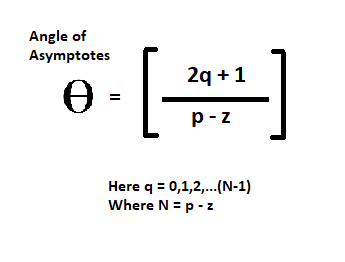Rule 4: Centroid : Meeting point of asymptotes on real axis is called as centroid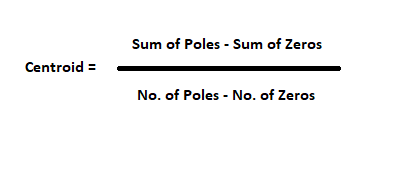Rule 5: Break Point (BP): There are two types

1. Break Away Point (BAP)
2. Break In Point (BIP)

Rule 6: Root locus intersection point (IP) with imaginary axis.

Rule 7:

a) Angle of departure: It is calculated for complex conjugated poles or imaginary poles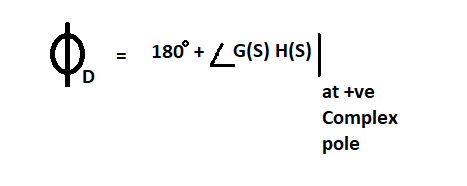b) Angle of  arrival: It is calculated for complex conjugate zeros or imaginary zeros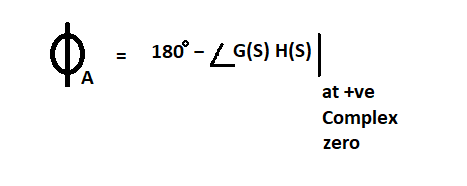Code :

 `% Row of 1×2``NUM = [1 10]; `` ` `% Row of 1×4``DEN = [1 6 8 0]; `` ` `% Row of 1×2``poly1 = [1 2]; `` ` `% Row of 1×2``poly2 = [1 4]; `` ` `% convolves vectors poly1 and poly2, ``% multiplying the polynomials whose coefficients ``% are the elements of poly1 and poly2``poly = conv(poly1, poly2);  `` ` `% returns the roots of the polynomial ``% represented by DEN as a column vector``roots(DEN); `` ` `% Continuous time transfer function``sys = tf(NUM, DEN);`` ` `% GUI for per-forming Root Locus analysis``rltool(sys); `

Output :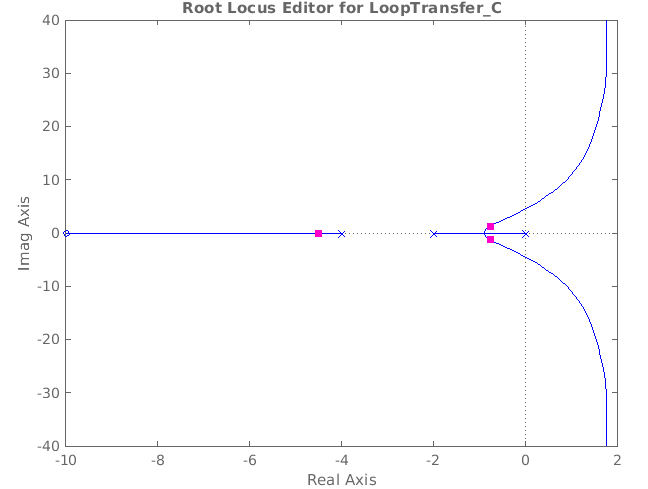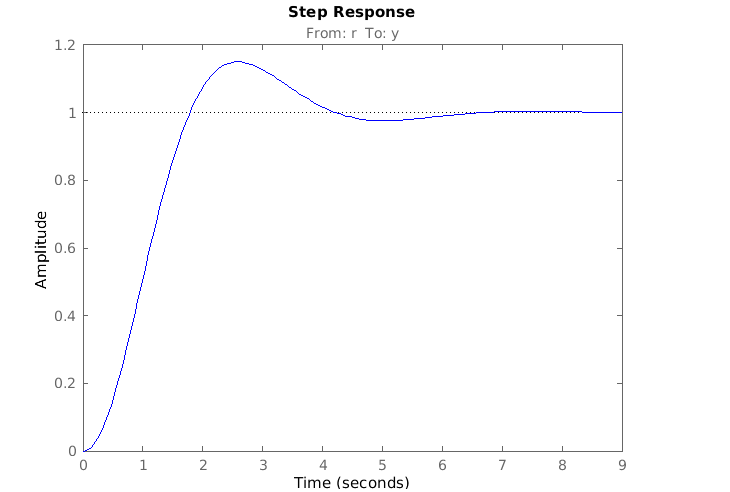My Personal Notes arrow_drop_up